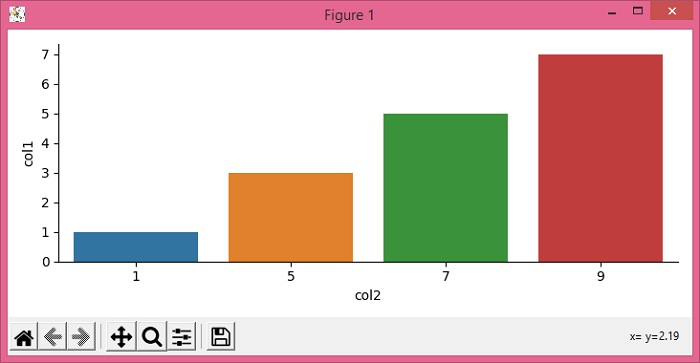# How to repress scientific notation in factorplot Y-axis in Seaborn / Matplotlib?

To repress scientific notation in factorplot Y-axis in Seaborn/Matplotlib, we can use style="plain" in ticklabel_format()method.

## Steps

• Set the figure size and adjust the padding between and around the subplots.

• Make a dataframe with keys, col1 and col2.

• The factorplot() has been renamed to catplot().

• To repress the scientific notation, use style="plain" in ticklabel_format() method.

• To display the figure, use show() method.

## Example

from matplotlib import pyplot as plt
import pandas as pd
import seaborn as sns

plt.rcParams["figure.figsize"] = [7.50, 3.50]
plt.rcParams["figure.autolayout"] = True

df = pd.DataFrame({"col1": [1, 3, 5, 7, 1], "col2": [1, 5, 7, 9, 1]})

sns.catplot(y="col1", x="col2", kind='bar', data=df, label="Total", height=3.5)

plt.ticklabel_format(style='plain', axis='y')

plt.show()

## Output